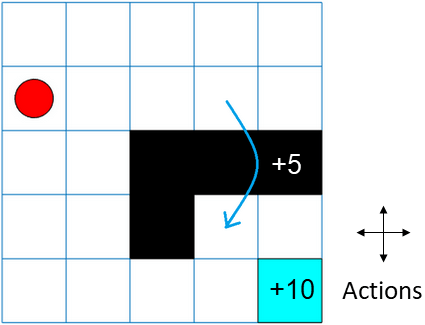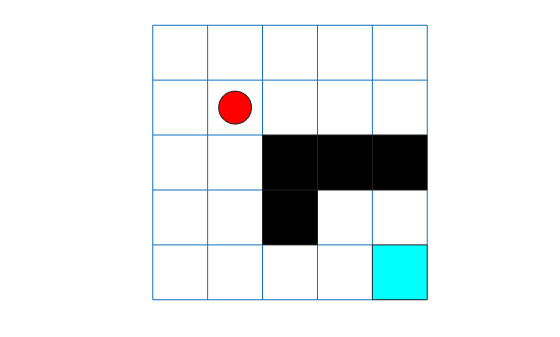createGridWorld

Create a two-dimensional grid world for reinforcement learning

Description

example

GW = createGridWorld(m,n) creates a grid world GW of size m-by-n with default actions of ['N';'S';'E';'W'].

GW = createGridWorld(m,n,moves) creates a grid world GW of size m-by-n with actions specified by moves.

Examples

collapse all

For this example, consider a 5-by-5 grid world with the following rules:

1. A 5-by-5 grid world bounded by borders, with 4 possible actions (North = 1, South = 2, East = 3, West = 4).

2. The agent begins from cell [2,1] (second row, first column).

3. The agent receives reward +10 if it reaches the terminal state at cell [5,5] (blue).

4. The environment contains a special jump from cell [2,4] to cell [4,4] with +5 reward.

5. The agent is blocked by obstacles in cells [3,3], [3,4], [3,5] and [4,3] (black cells).

6. All other actions result in -1 reward.First, create a GridWorld object using the createGridWorld function.

GW = createGridWorld(5,5)
GW =
GridWorld with properties:

GridSize: [5 5]
CurrentState: "[1,1]"
States: [25x1 string]
Actions: [4x1 string]
T: [25x25x4 double]
R: [25x25x4 double]
ObstacleStates: [0x1 string]
TerminalStates: [0x1 string]

Now, set the initial, terminal and obstacle states.

GW.CurrentState = '[2,1]';
GW.TerminalStates = '[5,5]';
GW.ObstacleStates = ["[3,3]";"[3,4]";"[3,5]";"[4,3]"];

Update the state transition matrix for the obstacle states and set the jump rule over the obstacle states.

GW.T(state2idx(GW,"[2,4]"),:,:) = 0;
GW.T(state2idx(GW,"[2,4]"),state2idx(GW,"[4,4]"),:) = 1;

Next, define the rewards in the reward transition matrix.

nS = numel(GW.States);
nA = numel(GW.Actions);
GW.R = -1*ones(nS,nS,nA);
GW.R(state2idx(GW,"[2,4]"),state2idx(GW,"[4,4]"),:) = 5;
GW.R(:,state2idx(GW,GW.TerminalStates),:) = 10;

Now, use rlMDPEnv to create a grid world environment using the GridWorld object GW.

env = rlMDPEnv(GW)
env =
rlMDPEnv with properties:

Model: [1x1 rl.env.GridWorld]
ResetFcn: []

You can visualize the grid world environment using the plot function.

plot(env)Input Arguments

collapse all

Number of rows of the grid world, specified as a scalar.

Number of columns of the grid world, specified as a scalar.

Action names, specified as either 'Standard' or 'Kings'. When moves is set to

• 'Standard', the actions are ['N';'S';'E';'W'].

• 'Kings', the actions are ['N';'S';'E';'W';'NE';'NW';'SE';'SW'].

Output Arguments

collapse all

Two-dimensional grid world, returned as a GridWorld object with properties listed below. For more information, see Create Custom Grid World Environments.

Size of the grid world, specified as a [m,n] vector.

Name of the current state, specified as a string.

State names, specified as a string vector of length m*n.

Action names, specified as a string vector. The length of the Actions vector is determined by the moves argument.

Actions is a string vector of length:

• Four, if moves is specified as 'Standard'.

• Eight, moves is specified as 'Kings'.

State transition matrix, specified as a 3-D array, which determines the possible movements of the agent in an environment. State transition matrix T is a probability matrix that indicates how likely the agent will move from the current state s to any possible next state s' by performing action a. T is given by,

T is:

• A K-by-K-by-4 array, if moves is specified as 'Standard'. Here, K = m*n.

• A K-by-K-by-8 array, if moves is specified as 'Kings'.

Reward transition matrix, specified as a 3-D array, determines how much reward the agent receives after performing an action in the environment. R has the same shape and size as state transition matrix T. Reward transition matrix R is given by,

R is:

• A K-by-K-by-4 array, if moves is specified as 'Standard'. Here, K = m*n.

• A K-by-K-by-8 array, if moves is specified as 'Kings'.

State names that cannot be reached in the grid world, specified as a string vector.

Terminal state names in the grid world, specified as a string vector.